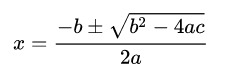EXPONENT RULES
GEOGRAPHY
EQUATIONS
BRANDS
GRAPHING
1

Simplify this expression:

3 7 · 3 7

314

1

This is the smallest of the 5 "Great Lakes"

Lake Erie

1

d = ?

7 + 9d = 7d + 3

d = -2

1

Name this brand: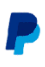Paypal

1

Determine the equation of the line from its graph:y = 4x + 8

1

Simplify this expression:

(24 ) 5

220

1

This is the mountain range that runs through Argentina, Chile, Bolivia, and Peru (and a few other countries).

The Andes

1

p = ?

3(1 + p) = −5(p + 1)

p = -1

1

Name this brand: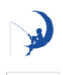Dreamworks

1

Determine the equation of the line from its graph:y = x − 6

2

6^3*6^0

SOLVE this expression:

216

2

This is the first African country you would enter if you traveled due south from Paris, France along the global longitude line.

Algeria

2

s = ?

5(1 + s) = −9s + 6

s=1/14

2

Name this brand: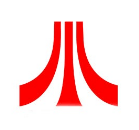Atari

2

Determine the equation of the line from its graph: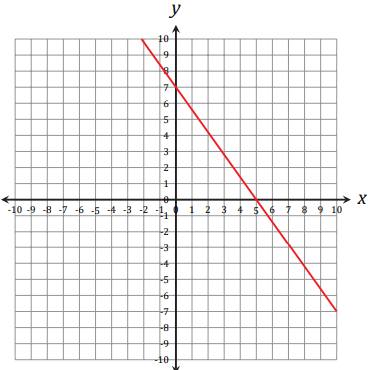y = − 7 /5 x + 7

3

Simplify this expression:

5^3/5^8

1/5^5

3

These are the 3 most populous cities (city proper) in Asia, in no particular order.

Tokyo, Japan

Delhi, India

Shanghai, China

3

q = ?

−2q − 3 = −2(2q + 1)

q=1/2

3

Name this brand: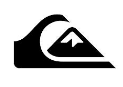Quiksilver

3

Determine the equation of the line from its graph: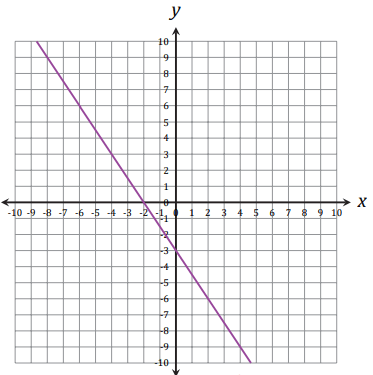y = − 3 /2 x − 3

4

Solve this equation using PEMDAS:

3^2+(4*4)(12)

201

4

This is the name of the city currently known as Istanbul, just before is was known as Constantinople (like, before Constantine conquered it in 324).

Byzantium

4

k = ?

−6k + 1 = −2 + 7k

k=3/13

4

Name this brand: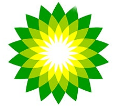BP (British Petroleum)

4

What is the name of this silliness: